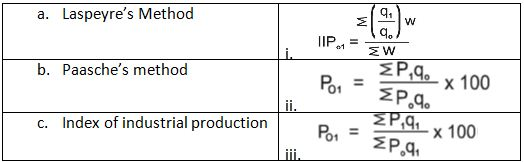# Introduction to Index Number - Online Test

Q1. The base year of IIP is
Explaination / Solution:

IIP is calculated by the Central Statistical Organisation (CSO). CSO takes the base year as 2004-05.

Q2. Laspeyre’s index formula uses the weights of the
Explaination / Solution:

In case of calculating the price index, assuming that for individual item price at the base period.

Q3. An appropriate method for working out consumer price index is
Explaination / Solution:

In this method, the family budgets of a large number of people are carefully studied and the aggregate expenditure of the average family on various items is estimated.

Q4. The weights used in Passche’ s formula belong to
Explaination / Solution:

Paasche’s Method: Under this method of calculating Price Index, the quantities of the current year are used as weights as compared to base year quantities used by Laspeyres.

Q5. _________ is known as Ideal index number
Explaination / Solution:

Fisher has discovered weighted index number in which he tries to calculate best index number because he has taken both current and past years quantity as the base of price index number.

Q6. Formula for calculating price index is
Explaination / Solution:
No Explaination.

Q7. Match the following. Options are as followsExplaination / Solution:

(i)     Laspeyre suggested that for the purposes of calculating Price Indices, the quantities in the base year should be taken as weights.
(ii)    Paasche’s method of calculating Price Index, the quantities of the current year are used as weights.
(iii)    The Index of Industrial Production (IIP) is an index for India which details out the growth of various sectors in an economy such as mining, electricity and manufacturing.

Q8. ______ are devices for measuring differences in the magnitude of a group of related variables
Explaination / Solution:

An index number is a statistical device for measuring changes in the magnitude of a group of related variables.

Q9. _____ in which current year quantities are used
Explaination / Solution:

Passche's Price Index is calculated by taking current year quantities as base therfore it is also called current year quantity weight method.

Q10. The main group of industries covered by IIP are
Explaination / Solution:

The Index of Industrial Production (IIP) is an index for India which details out the growth of various sectors in an economy such as mineral mining, electricity and manufacturing.